# Solving Word Problems by Adding and Subtracting Fractions and Mixed Numbers

## Learn How to Solve Fraction Word Problems with Examples and Interactive ExercisesExample 1: Rachel rode her bike for one-fifth of a mile on Monday and two-fifths of a mile on Tuesday. How many miles did she ride altogether?

Analysis: To solve this problem, we will add two fractions with like denominators.

Solution: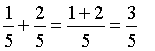Answer: Rachel rode her bike for three-fifths of a mile altogether.Example 2: Stefanie swam four-fifths of a lap in the morning and seven-fifteenths of a lap in the evening. How much farther did Stefanie swim in the morning than in the evening?

Analysis: To solve this problem, we will subtract two fractions with unlike denominators.

Solution: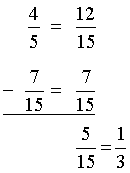Answer: Stefanie swam one-third of a lap farther in the morning.Example 3: It took Nick five-thirds of an hour to complete his math homework on Monday, three-fourths of an hour on Tuesday, and five-sixths of an hour on Wednesday. How many hours did he take to complete his homework altogether?

Analysis: To solve this problem, we will add three fractions with unlike denominators. Note that the first is an improper fraction.

Solution: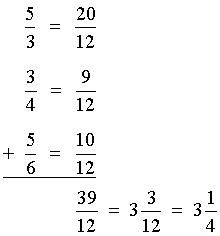Answer: It took Nick three and one-fourth hours to complete his homework altogether.Example 4: Dina added five-sixths of a bag of soil to her garden. Her neighbor Natasha added eleven-eighths bags of soil to her garden. How much more soil did Natasha add than Dina?

Analysis: To solve this problem, we will subtract two fractions with unlike denominators.

Solution: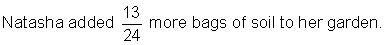Example 5: At a pizza party, Diego and his friends ate three and one-fourth cheese pizzas and two and three-fourths pepperoni pizzas. How much pizza did they eat in all?

Analysis: To solve this problem, we will add two mixed numbers, with the fractional parts having like denominators.

Solution: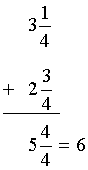Answer: Diego and his friends ate six pizzas in all.Example 6: The Cocozzelli family drove their car for five and five-sixths days to reach their vacation home, and then drove for six and one-sixth days to return home. How much longer did it take them to drive home?

Analysis: To solve this problem, we will subtract two mixed numbers, with the fractional parts having like denominators.

Solution: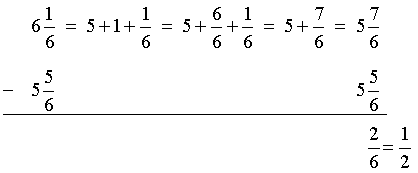Answer: The Cocozzelli family took one-half more days to drive home.Example 7: A warehouse has 12 and nine-tenths meters of tape in one area of the building, and eight and three-fifths meters of tape in another part. How much tape does the warehouse have in all?

Analysis: To solve this problem, we will add two mixed numbers, with the fractional parts having unlike denominators.

Solution: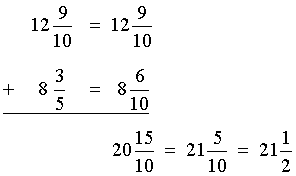Answer: The warehouse has 21 and one-half meters of tape in all.Example 8: An electrician has three and seven-sixteenths cm of wire. He needs only two and five-eighths cm of wire for a job. How much wire does he need to cut?

Analysis: To solve this problem, we will subtract two mixed numbers, with the fractional parts having unlike denominators.

Solution: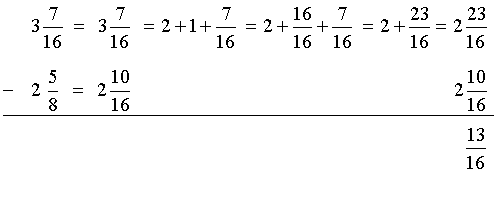Answer: The electrician needs to cut 13 sixteenths cm of wire.Example 9: A carpenter had a piece of wood that was 15 feet in length. If he needs only 10 and five-twelfths feet of wood, then how much wood should he cut?

Analysis: To solve this problem, we will subtract a mixed number from a whole number.

Solution: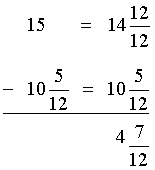Answer: The carpenter needs to cut four and seven-twelfths feet of wood.

Summary: In this lesson we learned how to solve word problems involving addition and subtraction of fractions and mixed numbers. We used the following skills to solve these problems:

1. Add fractions with like denominators.
2. Subtract fractions with like denominators.
3. Find the LCD.
4. Add fractions with unlike denominators.
5. Subtract fractions with unlike denominators.
6. Add mixed numbers with like denominators.
7. Subtract mixed numbers with like denominators.
8. Add mixed numbers with unlike denominators.
9. Subtract mixed numbers with unlike denominators.

### Exercises

Directions: Subtract the mixed numbers in each exercise below. Be sure to simplify your result, if necessary. Click once in an ANSWER BOX and type in your answer; then click ENTER. After you click ENTER, a message will appear in the RESULTS BOX to indicate whether your answer is correct or incorrect. To start over, click CLEAR.

Note: To write the fraction three-fourths, enter 3/4 into the form. To write the mixed number four and two-thirds, enter 4, a space, and then 2/3 into the form.

 1 A recipe needs 3/4 teaspoon black pepper and 1/4 red pepper. How much more black pepper is needed than red pepper for this recipe?   ANSWER BOX:   RESULTS BOX:
 2 One evening, a restaurant served a total of 1/2 of a loaf of wheat bread and 7/8 of a loaf of white bread. How many loaves were served in all?   ANSWER BOX:    RESULTS BOX:
 3 Robin and Kelly own neighboring cornfields. Robin harvested 4 and 3/10 acres of corn on Monday and Kelly harvested 2 and 1/10 acres. How many more acres did Robin harvest than Kelly?   ANSWER BOX:   acres   RESULTS BOX:
 4 Juanita needed 3 and 2/3 hours to take a standardized test, and Jordan needed 5 and 1/4 hours. How much more time did Jordan need than Juanita to take the test?   ANSWER BOX:   hours   RESULTS BOX:
 5 An airline agent checked in 10 and 1/3 kg of baggage for one passenger and another 8 and 5/6 kg of baggage for his travel companion. How many kilograms of luggage did the agent check in all?   ANSWER BOX:   kg    RESULTS BOX:
 Lessons on Adding and Subtracting Fractions and Mixed Numbers 1. Adding Fractions with Like Denominators 2. Subtracting Fractions with Like Denominators 3. Adding and Subtracting Fractions with Unlike Denominators 4. Adding Mixed Numbers 5. Subtracting Mixed Numbers 6. Solving Word Problems 7. Practice Exercises 8. Challenge Exercises 9. Solutions